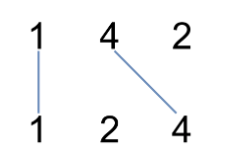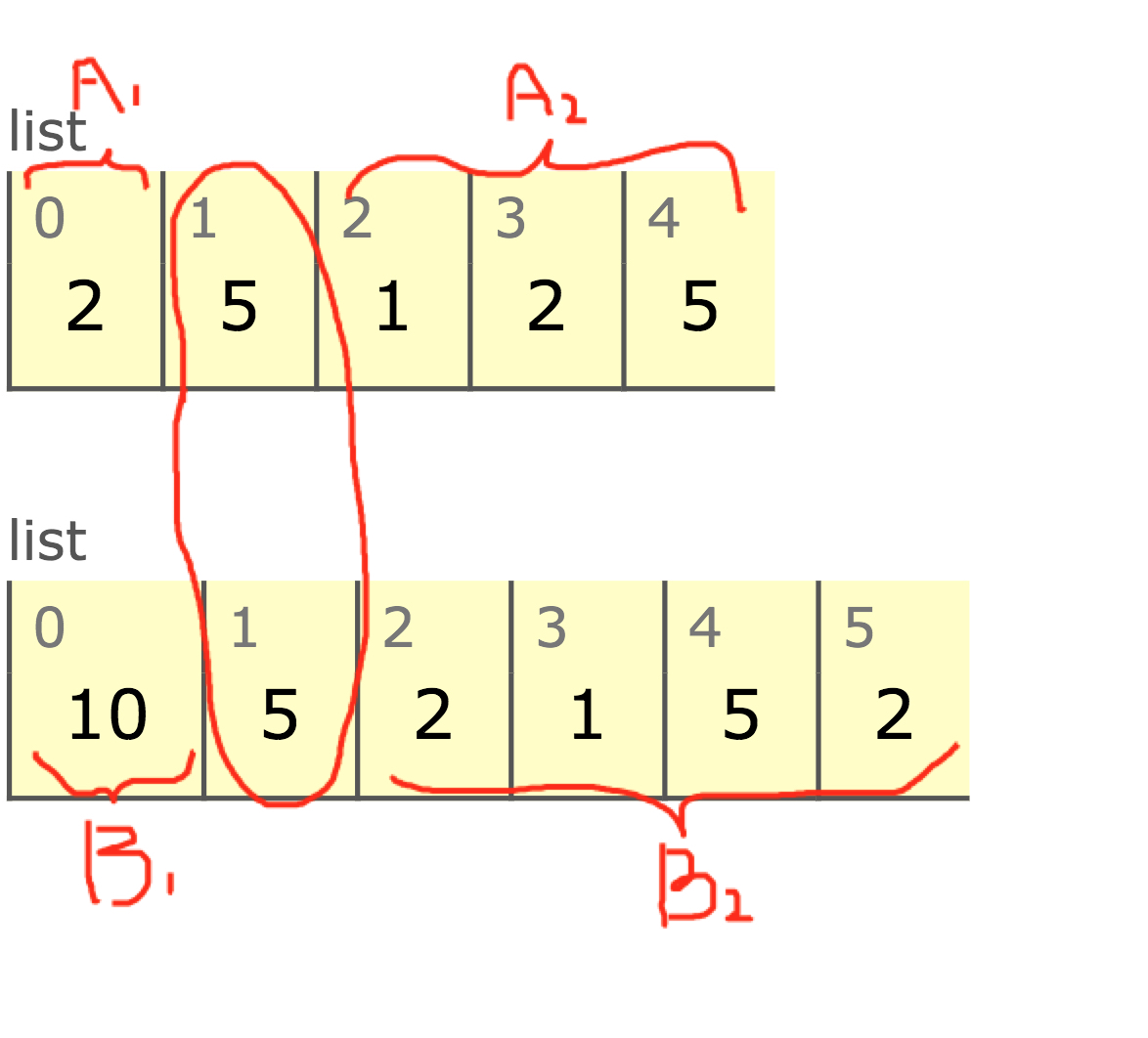# 1035. Uncrossed Lines

## Problem Setting¶

We write the integers of A and B (in the order they are given) on two separate horizontal lines.

Now, we may draw a straight line connecting two numbers A[i] and B[j] as long as A[i] == B[j], and the line we draw does not intersect any other connecting (non-horizontal) line.

Return the maximum number of connecting lines we can draw in this way.

### Example 1:¶Input: A = [1,4,2], B = [1,2,4]

Output: 2

Explanation: We can draw 2 uncrossed lines as in the diagram. We cannot draw 3 uncrossed lines, because the line from A=4 to B=4 will intersect the line from A=2 to B=2.

### Example 2:¶

Input: A = [2,5,1,2,5], B = [10,5,2,1,5,2]

Output: 3

### Example 3:¶

Input: A = [1,3,7,1,7,5], B = [1,9,2,5,1]

Output: 2

## Solution from hanshaohey¶

In :
class Solution(object):
def maxUncrossedLines(self, A, B):
"""
:type A: List[int]
:type B: List[int]
:rtype: int
"""
memo={}
la,lb=len(A),len(B)
def helper(i,j):
if i>=la or j>=lb:
return 0
if (i,j) in memo:
return memo[i,j]
if A[i]==B[j]:
ans=1+helper(i+1,j+1)
else:
ans=max(helper(i+1,j),helper(i,j+1))
memo[i,j]=ans
return ans
return helper(0,0)


This problem can be solved by "Recursive function" and "Dynamic Programming" approach to re-use the calculated intermediate result for the final result.

In the above example, helper is the recursive function to calculate the intermediate/final results at given conditions (i, j). The memo variable stores those intermediate results

### Tips to use a dictionary for dynamic programming¶

In :
# dictionary can be indexed by 2 dimentions and keys will be tubple
d = {}
d[1, 2] = 1
d[1, 3] = 2
d[3, 1] = 5
d

Out:
{(1, 2): 1, (1, 3): 2, (3, 1): 5}
In :
# dictionary can be indexed by N dimentions and keys will be tubple
d = {}
d[1, 2, 3] = 1
d[1, 3, 5] = 2
d[3, 1, 's'] = 5
d

Out:
{(1, 2, 3): 1, (1, 3, 5): 2, (3, 1, 's'): 5}

### Step-by-step understanding¶

The overall flow is as below:

1. Initial setup
2. Define the recursive function helper
3. Execute helper with (i, j) = (0, 0) as a starting point.

#### Initial setup¶

This step defines the variables for storing intermediate results as memo and gets the length of input lists as la and lb

# dictionary to store intermediate results for i, j
memo={}

# length of the list A and B
la,lb=len(A),len(B)


#### Define the recursive function helper¶

What we want to implement for a recursive function are as follows:

Input & Output

• the inputs are two indexes (i, j) of pointer on list A and B
• the output is the maximum number of uncrossed lines

Edge Cases

• Given 0 length of A and B
• (i, j) already reaches to the end index i.e., i==len(A), j ==len(B)

Internal Process

1. We would like to split the lists A and B when we find the same value in both list. In the example 2, first we will find 5 and draw the line.

2. Add +1 to the non crossed line count

3. Re-use the function for $A_2$ and $B_2$ as below.

4. On the other hand, if we do not find the same value, we will run two scenarios: move i to one index ahead or move j to one index ahead.

5. Compare the two scenario and take the larger number of non crossed lines.